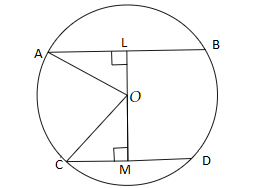# The lengths of two parallel chords of a circle are $6 \mathrm{~cm}$ and $8 \mathrm{~cm}$. If the smaller chord is at distance $4 \mathrm{~cm}$ from the centre, what is the distance of the other chord from the centre?

Given:

The length of two parallel chords of a circle are $6\ cm$ and $8\ cm$.

The smaller chord is at a distance of $4\ cm$ from the centre.

To do:

We have to find the distance of the other chord from the centre.

Solution:

Let a circle with centre $O$ and two parallel chords $AB$ and $CD$ are $AB = 6\ cm, CD = 8\ cm$

Let $OL \perp AB$ and $OM \perp CD$Therefore,

$OL = 4\ cm$

Let $OM = x\ cm$ and $r$ be the radius of the circle.

In right angled triangle $\mathrm{OAL}$,

$\mathrm{OA}^{2}=\mathrm{OL}^{2}+\mathrm{AL}^{2}$

$=4^{2}+(\frac{6}{2})^{2}$

$=16+9$

$=25$.......(i)

In right angled triangle $\Delta \mathrm{OMC}$,

$\mathrm{OC}^{2}=\mathrm{OM}^{2}+\mathrm{CM}^{2}$

$r^{2}=x^{2}+(\frac{8}{2})^{2}$

$=x^{2}+(4)^{2}$$=x^{2}+16$..........(ii)

From (i) and (ii), we get,

$x^{2}+16=25$

$\Rightarrow x^{2}=25-16=9$

$\Rightarrow x^{2}=(3)^{2}$

$x=3 \mathrm{~cm}$

Distance $=3 \mathrm{~cm}$

The distance of the other chord from the centre is $3\ cm$.

Updated on: 10-Oct-2022

26 Views